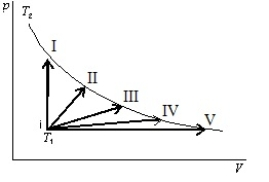# Fundamentals of Physics Study Set 2

Physics & Astronomy

## Quiz 20 :Entropy and the Second Law of ThermodynamicsLooking for Introductory Physics Homework Help?

## Quiz 20 :Entropy and the Second Law of Thermodynamics

Showing 1 - 20 of 61In a reversible process the system:
Free
Multiple Choice

AA slow (quasi-static)process is NOT reversible if:
Free
Multiple Choice

DPossible units of entropy are:
Free
Multiple Choice

BMultiple ChoiceFor all reversible processes involving a system and its environment:
Multiple ChoiceFor all irreversible processes involving a system and its environment:
Multiple ChoiceThe change in entropy is zero for:
Multiple ChoiceWhich of the following processes leads to a change in entropy of zero for the system undergoing the process?
Multiple ChoiceAn ideal gas expands into a vacuum in a rigid vessel.As a result there is:
Multiple ChoiceWhich of the following is NOT a state variable?
Multiple ChoiceA hot object and a cold object are placed in thermal contact and the combination is isolated.They transfer energy until they reach a common temperature.The change Sh in the entropy of the hot object, the change Sc in the entropy of the cold object, and the change Stotal in the entropy of the combination are:
Multiple ChoiceConsider all possible isothermal contractions of an ideal gas.The entropy of the gas:
Multiple ChoiceRank from smallest to largest, the changes in entropy of a pan of water on a hot plate, as the temperature of the waterMultiple ChoiceAn ideal gas is to taken reversibly from state i, at temperature T1, to other states labeled I, II, III, IV and V on the p-V diagram below.All are at the same temperature T2. Rank the five processes according to the change in entropy of the gas, least to greatest.Multiple ChoiceAn ideal gas, consisting of n moles, undergoes a reversible isothermal process during which the volume changes from Vi to Vf.The change in entropy of the thermal reservoir in contact with the gas is given by:
Multiple ChoiceOne mole of an ideal gas expands reversibly and isothermally at temperature T until its volume is doubled.The change of entropy of this gas for this process is:
Multiple ChoiceAn ideal gas, consisting of n moles, undergoes an irreversible process in which the temperature has the same value at the beginning and end.If the volume changes from Vi to Vf, the change in entropy is given by:
Multiple ChoiceThe temperature of n moles of a gas is increased from Ti to Tf at constant pressure.If the molar specific heat at constant pressure is Cp and is independent of temperature, then change in the entropy of the gas is:
Multiple Choice